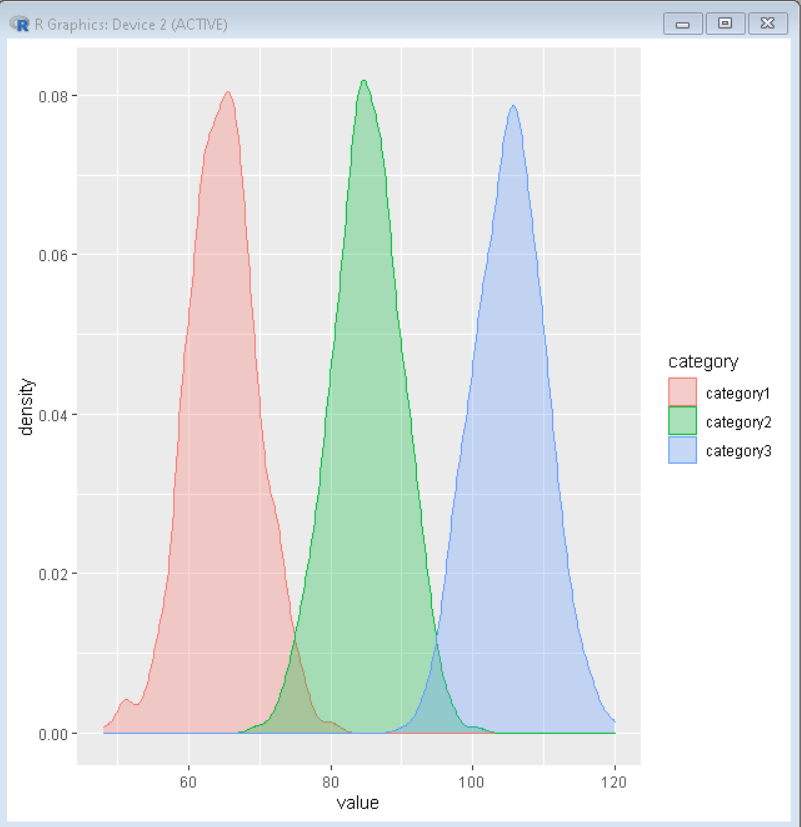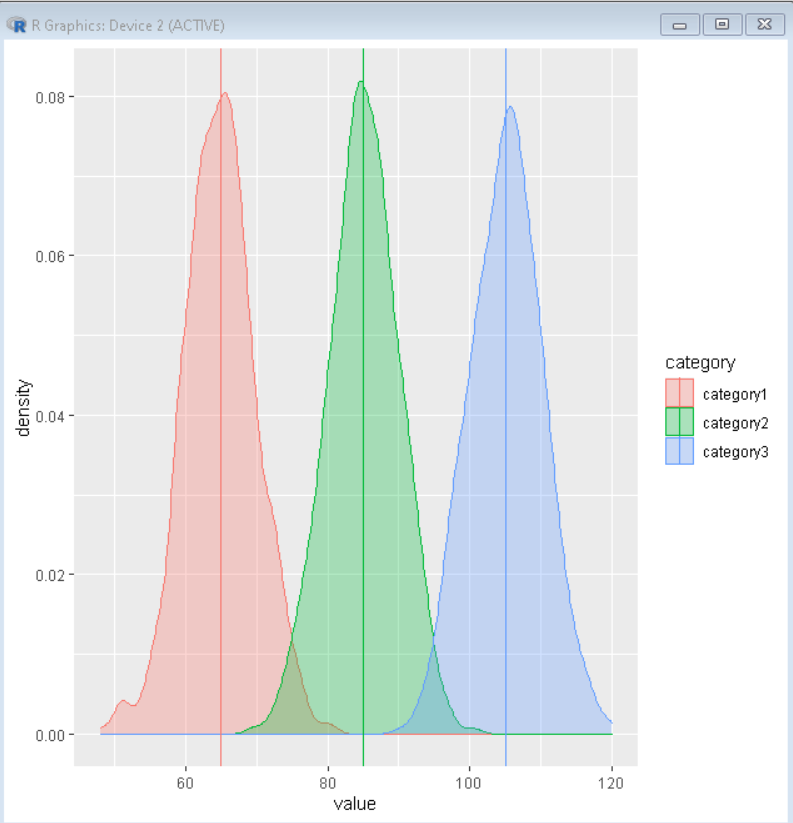# How to Add Vertical Lines By a Variable in Multiple Density Plots with ggplot2 in R

• Last Updated : 24 Oct, 2021

In this article, we will discuss how to add vertical lines by a variable in multiple density plots with ggplot2 package in the R  Programming language.

To do so first we will create multiple density plots colored by group and then add the line as a separate element.

## Basic Multiple Density Plot:

To make multiple density plots with coloring by variable in R with ggplot2, we firstly make a data frame with values and category. Then we draw the ggplot2 density plot using the geom_desnity() function. To color them according to the variable we add the fill property as a category in the ggplot() function.

Syntax:

ggplot(dataFrame, aes( x, color, fill)) + geom_density()

Example:

We get multiple density plots in the ggplot of two colors corresponding to two-level/values for the second categorical variable. If our categorical variable has n levels, then ggplot2 would make multiple density plots with n densities/color.

## R

 `# load library``library``(tidyverse)`` ` `set.seed``(1234)`` ` `# create the dataframe``df <- ``data.frame``(``    ``category=``factor``(``rep``(``c``(``"category1"``, ``"category2"``,``"category3"``),``                        ``each=1000)),``    ``value=``round``(``c``(``rnorm``(1000, mean=65, sd=5),``                  ``rnorm``(1000, mean=85, sd=5),``                 ``rnorm``(1000, mean=105, sd=5))))`` ` ` ` ` ` `# Basic density plot with custom color``# color property to determine the color of plot``# fill property to determine the color beneath plot``ggplot``(df, ``aes``(x=value, color=category, fill=category)) +``geom_density``(alpha=0.3)`

Output:## Adding Line by a variable

To add a line by a variable to plot create a new data frame median to a data frame that stores the median of values grouped by categories. Then use the geom_vline function to draw a line across that point colored by category of data.

Syntax:

plot + geom_vline( dataframe, aes( xintercept, color ), size)

Example:

Here, we have calculated the median of values grouped by category and stored it in a data frame named median. Then used geom_vline() function to draw a line across the plot at that point colored according to the category of data.

To create a Median data frame we use,

```median <- df %>%
group_by(category) %>%
summarize(median=median(value))```

Median data frame made from group_by and summarize function looks like:

```# A tibble: 3 x 2
category  median
<fct>      <dbl>
1 category1     65
2 category2     85
3 category3    105```

## R

 `# load library``library``(tidyverse)`` ` `set.seed``(1234)``df <- ``data.frame``(``    ``category=``factor``(``rep``(``c``(``"category1"``, ``"category2"``,``"category3"``), ``                        ``each=1000)),``    ``value=``round``(``c``(``rnorm``(1000, mean=65, sd=5),``                  ``rnorm``(1000, mean=85, sd=5),``                 ``rnorm``(1000, mean=105, sd=5))))`` ` ` ` `# create median data using above dataframe``# group_by function groups the data of same category``# summarize function with median``# argument calculates the median of value column``median <- df %>%``  ``group_by``(category) %>%``  ``summarize``(median=``median``(value))`` ` ` ` `# Basic density plot with custom color``# color property to determine the color of plot``# fill property to determine the color beneath plot``# geom_vline function draws the line across median ``# of each group``ggplot``(df, ``aes``(x=value, color=category, fill=category)) +``geom_density``(alpha=0.3)+``  ``geom_vline``(data = median, ``aes``(xintercept = median, ``                                       ``color = category), size=0.5)`

Output:My Personal Notes arrow_drop_up